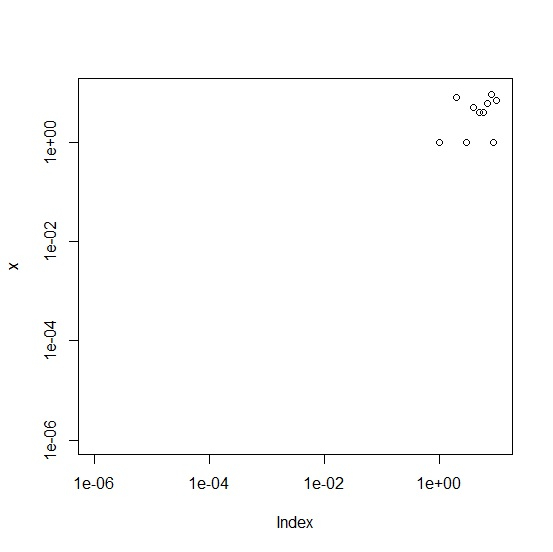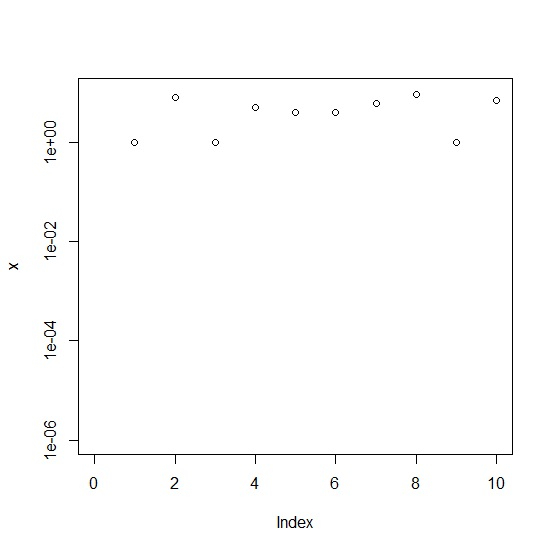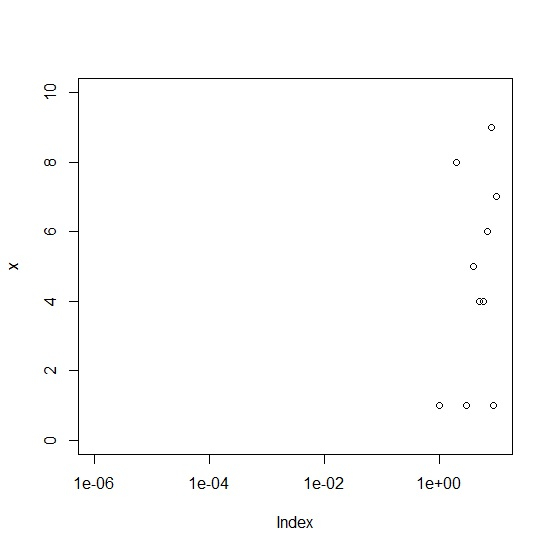# How to plot values with log scales on x and y axis or on a single axis in R?

We can plot numerical values in R with many scales and that includes log scale as well. Also, it is possible to plot the values with log scales on both the axes. In base R, the best way to do this is defining the axes values with decimal representation as shown in the below examples with well-defined log.

Consider the below vector −

## Example

Live Demo

set.seed(555)
x<-sample(1:9,10,replace=TRUE)
x

## Output

 4 8 7 5 6 1 9 2 1 8

Creating plot of x with log scale on both, x and y axis −

## Example

plot(x,xlim=c(0.000001,10),ylim=c(0.000001,10),log="xy")

## OutputCreating plot of x with log scale on y axis only −

plot(x,xlim=c(0.000001,10),ylim=c(0.000001,10),log="y")


## OutputCreating plot of x with log scale on x axis only −

plot(x,xlim=c(0.000001,10),ylim=c(0.000001,10),log="x")

## Output